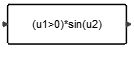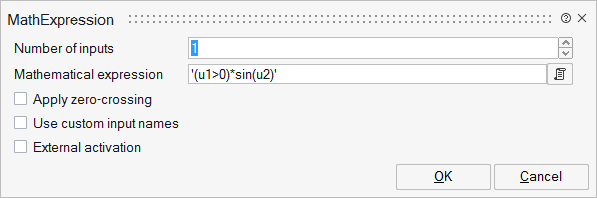# MathExpression

The output of this block is a scalar obtained by evaluating the mathematical expressions specified as block parameters. The expressions are given in terms of variables u1,...un (except if using custom input names) where ui is the ith scalar entry of the unique vector input or the ith scalar input in case the block has more than one input.MathOperations

## Description

The output of this block is a scalar obtained by evaluating the mathematical expressions specified as block parameters. The expressions are given in terms of variables u1,...un where ui is the ith scalar entry of the unique vector input or the ith scalar input in case the block has more than one input.

The expression may also include constant variables as long as they are defined in the diagram workspace. Only scalar operations are allowed between variables and input ui's. Variables however may be OML vectors or matrices; for example if A is an available matrix variable, the operation A(1,1)*u1 is allowed as expression.

Only double data type is allowed. Supported syntax is listed as following

Arithmetical operator: +, -, *, /, ^

Logical operator: |, &, !, ~

Relational operator: >, <, >=, <=, ==, ~=, <>

Elementary functions: abs, exp ,log, log10, min, max, sign, sqrt, hypot

Trigonometric functions: acos, acosh, asin, asinh, atan, atan2, atanh, cos, cosh, sin, sinh, tan

Rounding functions: ceil, floor, int (deprecated), fix, round

General math functions: rem power pow

## ParametersNameLabelDescriptionData TypeValid Values

nin

Number of inputs

Number of block inputs.

Number

work

Workspace Evaluation

used_names

Used names

Hidden parameter used by the block to indicate used environment variables.

exx

Mathematical expression

Mathematical expression that should be evaluated based on the inputs.

String

zc

Apply zero-crossing

Enables the detection of zero crossings.

Scalar

0
1

useinputnames

Use custom input names

Define input names different from the default. Default: unchecked.

Number

0
1

inputnames

Custom input names

The variable names of inputs.

Structure

inputnames/inputname

Variable name

Input variable name.

Cell of strings

externalActivation

External activation

Specifies whether the block receives an external activation or inherits its activation through its regular input ports. When External Activation is selected, an additional activation port is added to the block. By default, external activation is not selected.

Number

0
1

## Ports

NameTypeDescriptionIO TypeNumber

Port 1

explicit

output

1

Port 2

explicit

input

nin

Port 3

activation

input

externalActivation

NameValueDescription

always active

no

direct-feedthrough

yes

zero-crossing

depends on block parameters

mode

depends on block parameters

continuous-time state

no

discrete-time state

no